CS280 "Computer Vision"

Homework 1, Feb-2002.

Siddharth JainĀ, Cheng Chang ,ĀAlmudena Konrad
( morpheus@eecs.berkeley.educchang@eecs.berkeley.edualmudena@cs.berkeley.edu )

Written items

### 1. An absorbing medium.

• Expression for the radiance transferred from one surface patch of small area (dA) to another of small area in the presence of this medium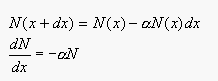(Equation 1)

or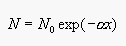(Equation 2)

which is the expression for the radiance transferred from one surface patch to another separated by a distance of x.

• Distribution of light.

• If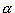is a very small positive number, the distribution of light falls off slowly.
• Ifis a medium sized positive number, the distribution of light falls off more rapidly than in the previous case.
• Ifis a large positive number, the distribution of light falls off very rapidly.

Consider a cube with source at the center of a face. Let d be the distance from the source to the corners of the opposite face. Then for a small fall off, let's say that 90% of the light is able to reach the corners at the opposite face. Applying Equation 2, we obtain: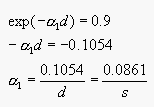where,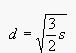and s represents the side of the cube.

For a medium sized fall off let's say 50% of the light is able to reach the corners, applying Equation 2, we obtain: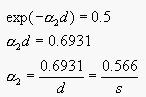And for a large fall off let's say only 10% of the light is able to reach the corners, we obtain: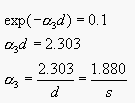Figure 1.1 illustrates the exponential decay of.

### 2. Lambertian nor specular surface.

A CD surface acts as a diffraction grating, separating white light into all the rainbow colors. An interesting phenomenon is the change of colors caused by the CD as we tilt the surface back and forth. Light waves reflected from the CD surface overlap and interfere with each other producing this coloring effect. This is known as interference. For a given viewing angle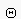, constructive interference occurs such that the rays from adjacent tracks each travel an extra distance of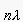, where is n is an integer that denotes the order of the image (see to Equation 3)(Ref ). Changing the angle, causes different wavelengths of light to constructively interfere. When the viewing angle is small, shorter wavelengths such as blue, violet, and indigo constructively interfere. At larger viewing angles, the longer wavelengths such as red, orange, and yellow constructively interfere (Ref ).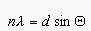(Equation 3)
where d is the grating constant and it represents the track spacing in the CD.

Examples of biological surfaces that show similar properties are insect wings (such as butterflies wings), hummingbird throat feathers, and organisms having scales on their skin like snakes and fish - the scales act as the diffraction grating.

### 3. Volume color.

For the answer to this question we go back to Equation 2 in Problem first. The translucent material, in this case the glass of wine, is a uniform absorbing medium. A glass of red wine absorbs all the wavelength strongly except the red wavelength. Furthermore, the red wavelength component also decays exponentially, as showed in Equation 2, where N represents the observed intensity, N0 is the original light intensity,is the absorption coefficient and x is the distance traveled through the medium.

A small glass of sufficiently deeply colored red wine looks black because the high concentration of colored pigment compensates for the small distance traveled by the light. We have an exponential attenuation of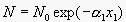.

A big glass of lightly colored red wine appears black because even though the absorbance is low due to low concentration of colored pigment, the distance is high because there is more wine in the glass. We have an exponential attenuation of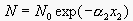.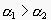but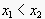, therefore the attenuation in both cases is relatively the same so the wine appears black in both glasses.

### 4. Scattering of light by air.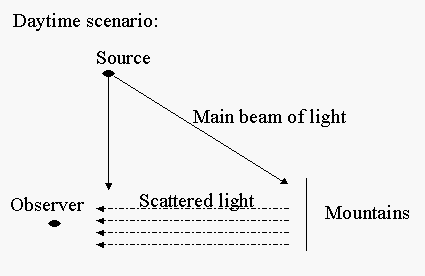During daytime the sunlight is scattered by the air molecules producing a blur between the observer and the mountains. We see the mountains because of the reflected and scattered light - so they appear as blurred patches of low intensity.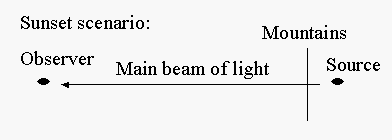We see the mountains because of the main beam of light so they are much sharper and more visible. Note that light suffers some attenuation in the air due to moisture.

Also note that at sunset an object overhead could appear blurred just as the mountains appeared blurred in the daytime. We estimate the distance for which air can be modeled as vacuum to be a few Kilometers, (<10) .

Practical exercises

### 2. Illuminant and surface reflectance.

According to our observation, the most significant error occurs in the light with low Wavelength (thus high frequency light). The possible reason is that the color dataset we used has a larger variance in low wavelength. In Figure 2.1, with 5 principal components, Ā Figure 2.2, with 7 principal components, Ā Figure 2.3, with 10 principal components, Ā Figure 2.4, with 12 principal components, Ā and Figure 2.5, with 20 principal components, the Y_coordinates are the errors (Euclidean distance from EXACT spectra to PCA spectra) the X_coordinates are the wavelengths (from 380 nm to 800 nm).

### 4. Human color constancy.

Human color constancy is the mechanism by which humans see colors. It refers to the lack of change in the perceived color of a surface as the illumination changes. The Human Visual System does not exhibit perfect color constancy. It also performs poorly in lighting with abnormal spectral content such as fluorescent light . There are a few researchers looking at the color constancy problem. For example, D'Zmura and Iverson in 1998, introduced a new approach to this problem which emphasizes the recovery of the spectral properties of light sources and surfaces that combine to produce the reflected lights that reach the eye. This approach relies on bilinear models of the visual response to reflected lights, and our first aim in this document, after a brief review of color constancy, is to describe such bilinear models .

### 5. An area source approximated as a grid of point sources.

1. The quantization errors is a direct consequence of the discretization of the area source giving a finite number of grid points. This means that if we are at a position P1 and suppose n points are visible and m are invisible, then there is some distance dmin such that if I move a distance less than dmin, m and n do not change - which is the approximation we make and which gives the quantization errors.

2. Figure 1Ā( parameters), illustrates an area source of approximately 12x12 grid, Figure 2Ā( parameters), illustrates an area source of approximately 25x25 grid, and Figure 3Ā( parameters), illustrates an area source of approximately 36x36 grid. From these figures, it is apparent that as the number of point sources goes up, the quantization error goes down (i.e., the image become more realistic and accurate).

3. If we change the geometry keeping the number of points in the source the same, then we can increase dmin. Figure 4Ā( parameters),Ā Figure 5Ā( parameters),Āand Figure 6Ā( parameters), show this effect. In these figures the occluder is tilted at an angle of 45 degrees.

Thus, we find that Figure 1, gives an image which is more accurate than the corresponding image for Figure 4, because dmin has increased.

#### References

1. http://fusioned.gat.com/Teachers/Curriculum/Curriculum-HTML/T03S-CD-diffract.html